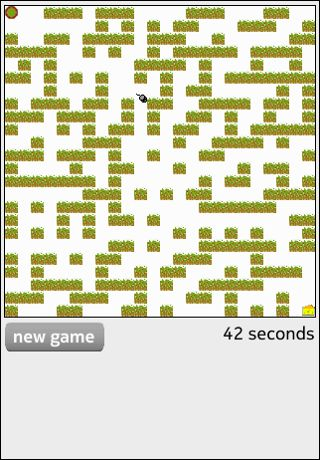# Qt Sensors - Maze QML Example

The Maze example demonstrates the TiltSensor QML type.##### Maze in QML

To write a QML application that will use the TiltSensor QML sensors type you need to do the following steps:

Import the QtSensors 5.x declarative plugin:

``` import QtSensors 5.0
```

In this example we use the TiltSensor with values based in degrees and an accuracy of 5 degree:

```     TiltSensor {
id: tiltSensor
active: true
}
```

Starting the sensor can be done by setting the 'enabled' property to true:

```         onTriggered: {
if (!tiltSensor.enabled)
tiltSensor.active = true;
```

The mouse should move by a factor of the tilt value:

```                 var xstep = 0;
xstep = tiltSensor.reading.yRotation * 0.1 //acceleration

var ystep = 0;
ystep = tiltSensor.reading.xRotation * 0.1 //acceleration
```

The walk direction of the mouse takes into account some collision detection:

```                 if (xstep < 1 && xstep > 0)
xstep = 0
else if (xstep > -1 && xstep < 0)
xstep = 0

if (ystep < 1 && ystep > 0)
ystep = 0;
else if (ystep > -1 && ystep < 0)
ystep = 0;

if ((xstep < 0 && mouseCtrl.x > 0
&& Lib.canMove(mouseCtrl.x + xstep,mouseCtrl.y))) {
xval = mouseCtrl.x + xstep;

} else if (xstep > 0 && mouseCtrl.x < (Lib.cellDimension * (Lib.dimension - 1))
&& Lib.canMove(mouseCtrl.x + xstep,mouseCtrl.y)) {
xval = mouseCtrl.x + xstep;
} else
xval = mouseCtrl.x;

if (ystep < 0 && mouseCtrl.y > 0
&& Lib.canMove(mouseCtrl.x, mouseCtrl.y + ystep)) {
yval = mouseCtrl.y + ystep;
} else if (ystep > 0 && (mouseCtrl.y < (Lib.cellDimension * (Lib.dimension - 1)))
&& Lib.canMove(mouseCtrl.x, mouseCtrl.y + ystep)) {
yval = mouseCtrl.y + ystep;
} else
yval = mouseCtrl.y

mouseCtrl.move(xval, yval);
```

The rotation of the mouse image is determined according to the angle that the mouse is moving.

```         var a = newy - mouse.y
var b = newx - mouse.x
angle = Math.atan2(-b, a) * mouse.radians_to_degrees
if (angle < 0)
angle = 360 + angle

img.rotation = angle
mouse.x = newx;
mouse.y = newy;
```

Example project @ code.qt.io